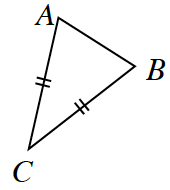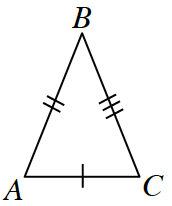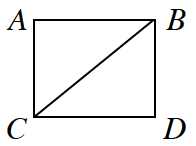### Home > INT2 > Chapter 1 > Lesson 1.1.2 > Problem1-17

1-17.

If no sides of a triangle have the same length, the triangle is called scalene (pronounced SKAY-leen). And, as you might remember, if the triangle has at least two sides that are the same length, the triangle is called isosceles. Use the markings or description in each diagram below to decide if $∆ABC$ is isosceles or scalene. Assume the diagrams are not drawn to scale.

Sides with the same markings mean the lengths are the same.

1.isosceles

1.scalene

1. $ABDC$ is a squareisosceles